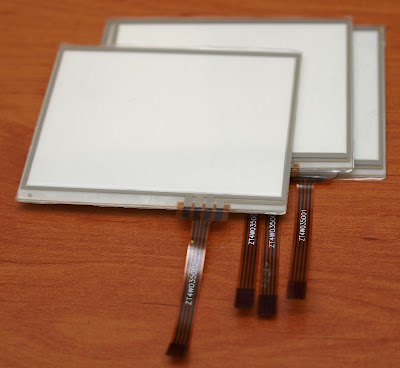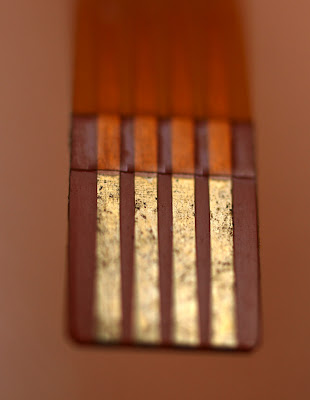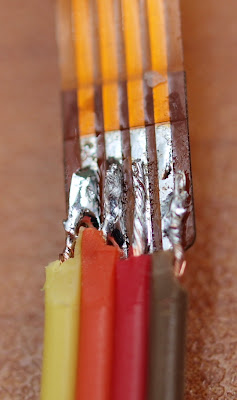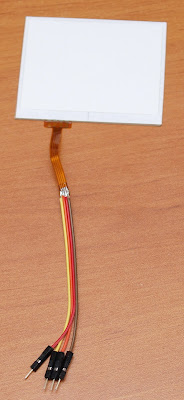## zaterdag 22 februari 2014

### Resistive Touch panel with ArduinoThese touch screens are easy to find, cheap and very easy to control.

Actually, I found that you don't even need any library if you plan to connect them to an Arduino. Just connect the four wires to four analog inputs and write some lines of code. It's pretty straight-forward.Actually, most effort was spent on soldering the tiny pads to standard jumper wires so I can actually do something with it. It's not the most pretty sight, but I got them connected and it works. Then, all it takes is plugging in the four wires into four analog pins on your Arduino. Yes, really, that's all.

Well, you do need some code to write of course. But these things are pretty easy to understand hence easy to code.To make things easy, let's give these borders a name. The screen consists of two layers on top of each other. When you press the screen, the layers connect at a certain point. This point can be measured in two steps: measure the X-value and the Y-value.To try them out, you could connect these to any DC power source and see what happens with a standard multimeter. Watch the movie below to see how I did this.

To measure the X-value, you should connect X1 to +5V and X2 to GND. Now, if you press the screen at a certain point, a voltage divider is created across the X-axis. Then, you can either measure the voltage on Y1 or Y2, that doesn't make any difference. The resistance of my total X-axis was approx. 700 Ohm, but that has no influence other than consuming a bit of current.

Same trick for the Y-axis. Connect Y1 to ground and Y2 to +5V (or any positive DC voltage) and measure the voltage on pins X1 or X2 (compared to ground of course).

Check this little video to see it working.
** by the way, the servos are optional and my hand is not shaky, the servos are ;-)

Connecting the panel to an Arduino is easy. Just connect all four pins to four analog inputs.

To check X:
- define X1 and X2 as OUTPUTS
- define Y1 and Y2 as INPUTS
- set X1 to HIGH and X2 to LOW
- use analogRead() on Y1 (or Y2) to get the value. This value will be a value between 0 and 1023, indicating the X-position of the pressed screen.

(note that I use HIGH left and LOW right of the panel, it was easy for my tests but this means the higher values are on the left of the screen)

To check Y:
- define Y1 and Y2 as OUTPUTS
- define X1 and X2 as INPUTS
- set Y1 to LOW and Y2 to HIGH
- use analogRead() on X1 (or X2) to get the value. This value will be a value between 0 and 1023, indicating the Y-position of the pressed screen.

These two functions will do the trick if you connect the pins to A0..A3
(X1 = A3, Y1 = A2, X2 = A1, Y2 = A0)

int xcoor(){
pinMode(A1,OUTPUT);
pinMode(A3,OUTPUT);
pinMode(A0,INPUT);
pinMode(A2,INPUT);
digitalWrite(A1,LOW);
digitalWrite(A3,HIGH);

}

int ycoor(){
pinMode(A0,OUTPUT);
pinMode(A2,OUTPUT);
pinMode(A1,INPUT);
pinMode(A3,INPUT);
digitalWrite(A0,LOW);
digitalWrite(A2,HIGH);